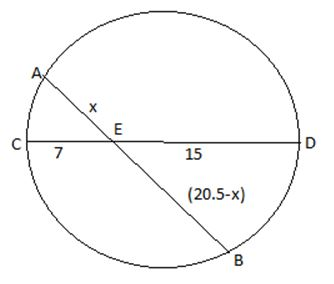# In a circle of radius 11 cm, CD is a diameter - Quantitative Aptitude – Geometry | Circles |

## CAT 2019 – Slot 1 - CAT Circles - Question 5 - In a circle of radius 11 cm, CD is a diameter

Q. 5: In a circle of radius 11 cm, CD is a diameter and AB is a chord of length 20.5 cm. If AB and CD intersect at a point E inside the circle and CE has length 7 cm, then the difference of the lengths of BE and AE, in cm, is

1.   2.5
2.   3.5
3.   0.5
4.   1.5

As per question following figure can be drawn,As we know AE*BE = CE*DE

X(20.5 –x) = 7*15 = 105

20.5x –x^2 = 105

x^2 -20.5x + 105 = 0

x^2 -10.5x – 10x +105 =0

(x -10)*(x-10.5) =0

So x = 10 or 10.5

Thus if AE = 10 then BE = 10.5 and vice versa

Required difference = (10.5 -10) = 0.5

### Past Year Question Paper & SolutionsCounselling Session
By IIM Mentor

#### Free Material Area